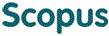### BROWSE

9 655

Cited 0 times in한국 성인 남자 긴뼈와 키의 상관관계

Other Titles
Correlation between the length of the long bone and the postmortem height of the Korean adult male
Authors
최병영
Issue Date
1993
Description
의학과/석사
Abstract
[한글] 긴뼈의 길이가 키와 상관관계가 있다는 사실이 알려져 있었다. 이를 근거하여 키를 추정할 수 있는 희귀식이 각 종족별 보고되어 있으나 한국인을 대상으로 한 공식은 없다. 사망 후 키를 계측하여 기록한 후 연세대학쿄 의과대학 해부학교실에 보완중인 한국인 성인 남자 시체 57구로부터 나온 긴뼈(상환골. 요골,척골, 대퇴골, 경골, 비골)를 대상으로 최대길이 및 대퇴골경사길이와 경골관절사이길이를 게측하였다. 자료로 단순희귀분석 및 증희귀분석을 한 결과 각각의 긴뼈와 키의 상관계수와 키를 추정할 수 있는 희귀식을 구하였다. 1. 한국인 성인 남자 시체의 사망 후 측정한 키의 범위는 147 - 178 cm, 평균은 163.1 cm 표준편차는 7.1 이였다. 2. 사망시 나이의 범위는 20 - 86세, 평균은 52.3세, 표준편차는 16.6였다. 3. 오른쪽과 왼쪽뼈의 길이는 유의한 차이를 보이지 않았다. 4. 모든 항목에서 키에 대한 상관계수는 0.6 이상이었다. 5. 키에 대하여 가장 높은 상관계수를 나타낸 항목은 대퇴골최대길이였으며 가장 낮은 상관계수를 나타낸 항목은 척골최대길이였다. 6. 긴뼈들간에 서로의 상관계수가 가장 낮은 항목은 비골최대길이와 상완골최대길이였다. 7. 대퇴골과 경골은 최대길이가 각각 경사길이, 관절사이길이보다 키에 대한 상관계수는 컸으나 통계적 차이를 보이지 않았다. 8. 단순희귀분석을 통한 희귀식중 설명력이 가장 높은 항목은 대퇴골최대길이였다 9. 중희귀분석을 이용하여 구한 희귀식중 가장 타당한 희귀식은 대퇴골최대길이와 상완골최대길이의 조합에 의한 희귀식이였다. 10. 희귀계수는 모든 희귀식애서 50%이상의 설명력(결정개수)을 보였다. Correlation between the length of the long bone and the postmortem height of the Korean adult male Byoung Young Choi Department of Medical Science, The Graduate School Yonsei University (Directed by Professor In Hyuk Chung) After measuring the postmortem height of 57 Korean male cadavers, the maximal length of long bones(humerus, radius, ulna, femur. tibia, fibula),the oblique length of femur. and the ordinarly length(interarticular length)of tibia. which were remained after dissection, were measured on osteometric board. And the intercorrelation matrices of each long bone length with height and regression equations on each long bones were gained. The results were as follows; 1. The range, mean and standard deviation of potmortem height of 57 Korean male adavers were 147.0 - 178.0 cm, 103,1 cm. 7.1,respectively. 2. The range. mean and standard deviation of age at the time of death were 20 - 86 yeah-old, 52.3 yeah-old. 16.6, respectively. 3. There was no statistical difference between all the pairs of right and left long bone. (P>0.05) 4. It was noted that the correlations coefficient of all variables was above 0.6. 5. It was the maximal length of femur which showed the highest correlation coefficient with height.(0.798) 6. It was the maximal length of ulna which showed the lowest correlation coefficient with height.(0.710) 7. It was the maximal length of humerus and fibula which showed the lowest correlation coefficient between the long bones.(0.648). 8. R squares of all regression equations were above 0.5. 9. There was no statistical significance between the regression equations of femur maximal length oblique length and intercondylar length. 10. It was more reliable to adapt the repression equation of multiple regression analysis those of single regression analysis. 11. It was noted that the regression equations of this study would be more reliable to adapt to Korean male then foreign regression equation.
[영문] After measuring the postmortem height of 57 Korean male cadavers, the maximal length of long bones(humerus, radius, ulna, femur. tibia, fibula),the oblique length of femur. and the ordinarly length(interarticular length)of tibia. which were remained after dissection, were measured on osteometric board. And the intercorrelation matrices of each long bone length with height and regression equations on each long bones were gained. The results were as follows; 1. The range, mean and standard deviation of potmortem height of 57 Korean male adavers were 147.0 - 178.0 cm, 103,1 cm. 7.1,respectively. 2. The range. mean and standard deviation of age at the time of death were 20 - 86 yeah-old, 52.3 yeah-old. 16.6, respectively. 3. There was no statistical difference between all the pairs of right and left long bone. (P>0.05) 4. It was noted that the correlations coefficient of all variables was above 0.6. 5. It was the maximal length of femur which showed the highest correlation coefficient with height.(0.798) 6. It was the maximal length of ulna which showed the lowest correlation coefficient with height.(0.710) 7. It was the maximal length of humerus and fibula which showed the lowest correlation coefficient between the long bones.(0.648). 8. R squares of all regression equations were above 0.5. 9. There was no statistical significance between the regression equations of femur maximal length oblique length and intercondylar length. 10. It was more reliable to adapt the repression equation of multiple regression analysis those of single regression analysis. 11. It was noted that the regression equations of this study would be more reliable to adapt to Korean male then foreign regression equation.
Full Text
https://ymlib.yonsei.ac.kr/catalog/search/book-detail/?cid=CAT000000007987
Files in This Item:
제한공개 원문입니다.
Appears in Collections:
1. College of Medicine (의과대학) > Others (기타) > 2. Thesis
URI
https://ir.ymlib.yonsei.ac.kr/handle/22282913/117184
사서에게 알리기